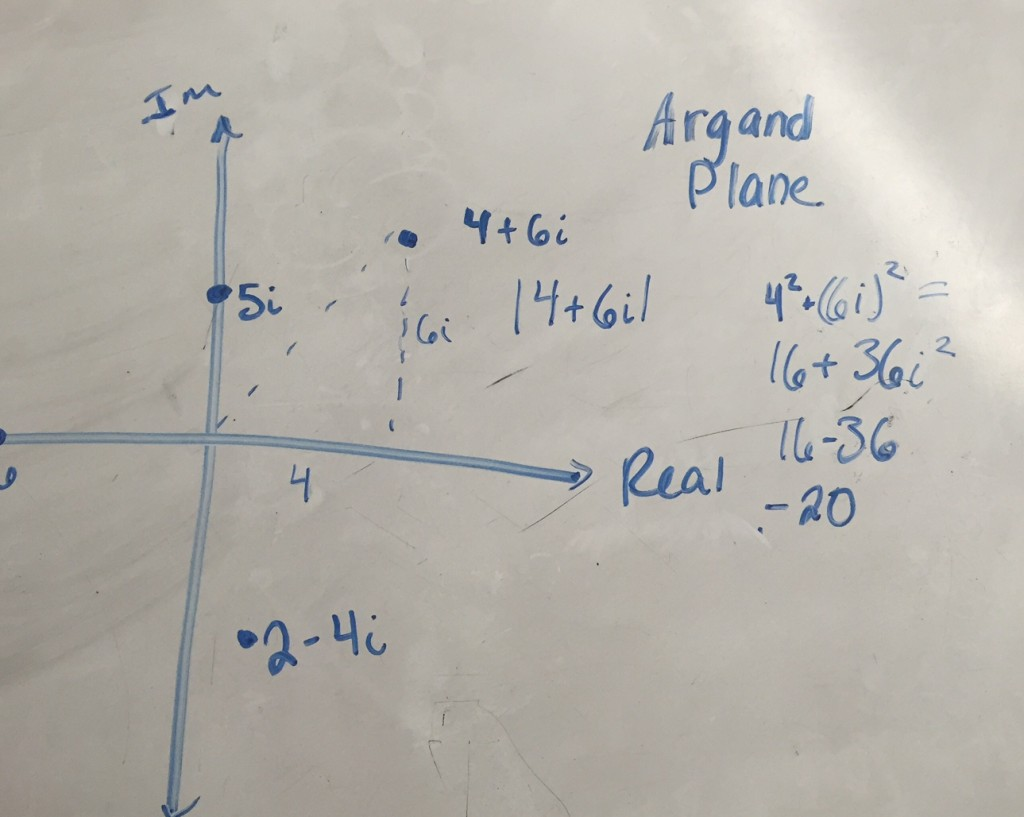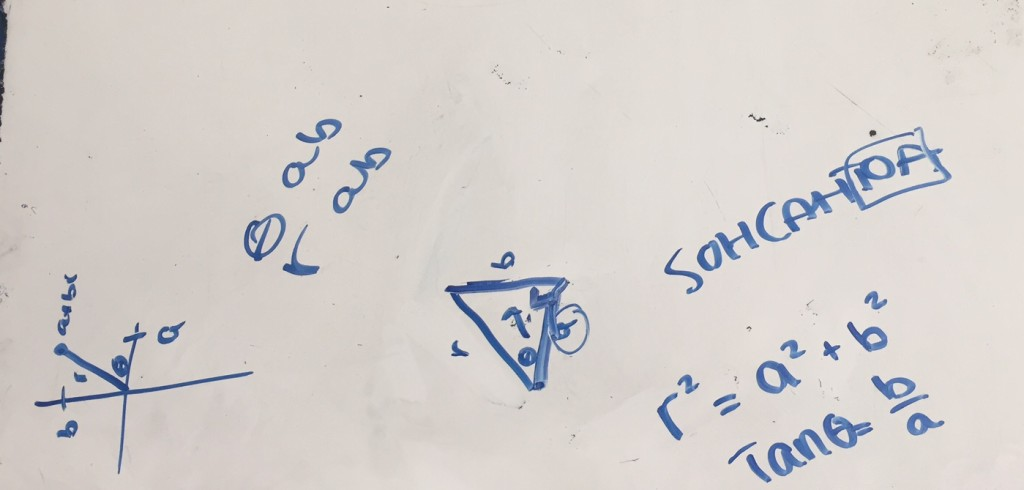This is all from day 2 of the Mandelbrot Fractal lesson.

A nice mistake here when the student was trying to find the size of this complex number. Can you spot it? Pretty common mistake at the beginning:Here’s some whiteboard work while they were just introduced to the polar form of a complex number. The question was “Can you write an equation with a, b, and r in it? Can you write an equation with a, b, and theta in it?”